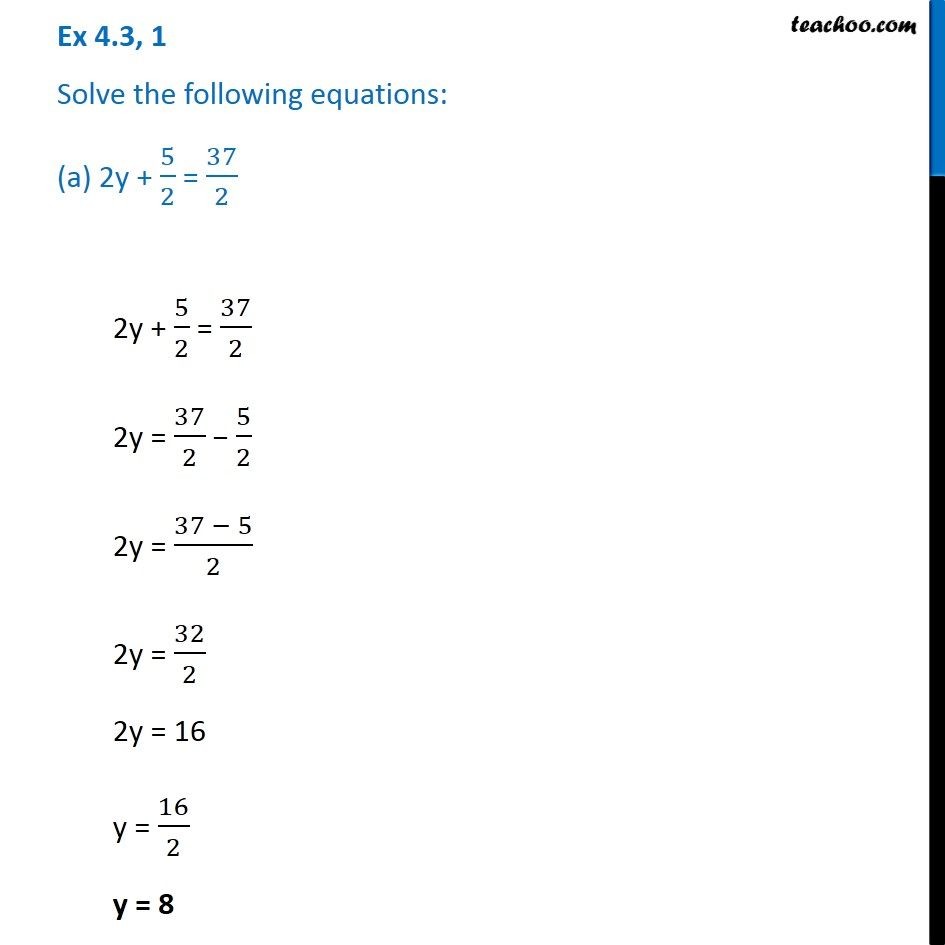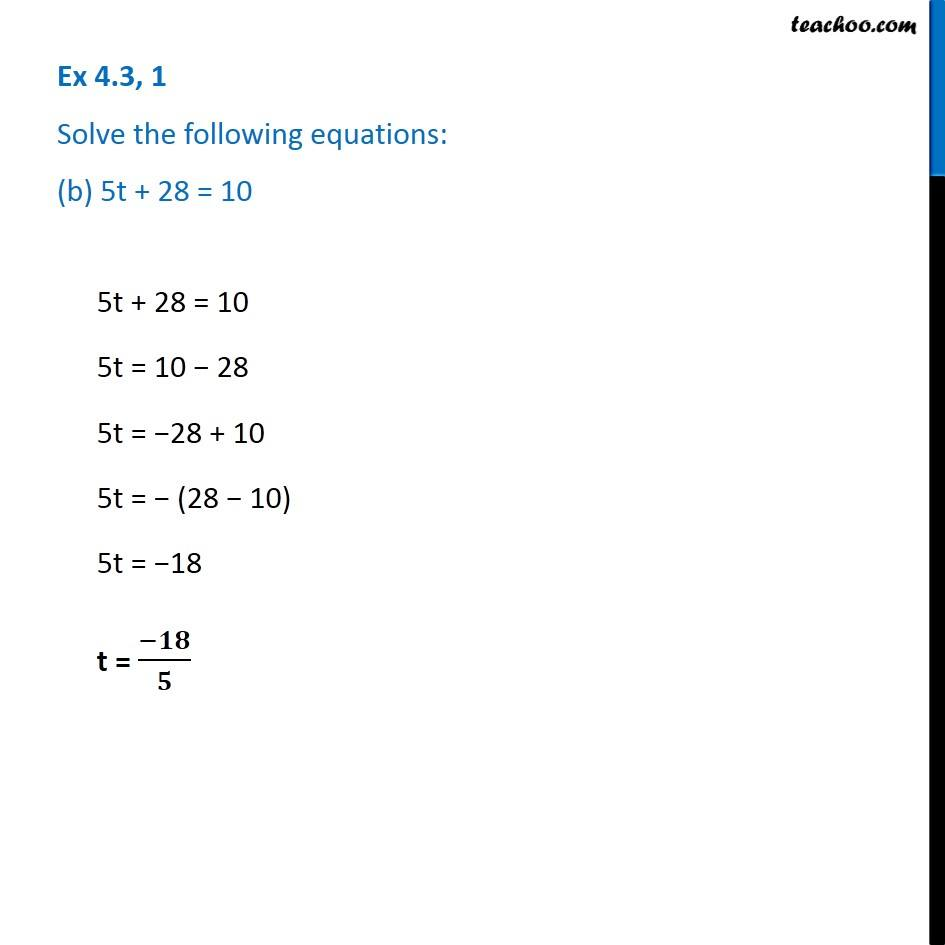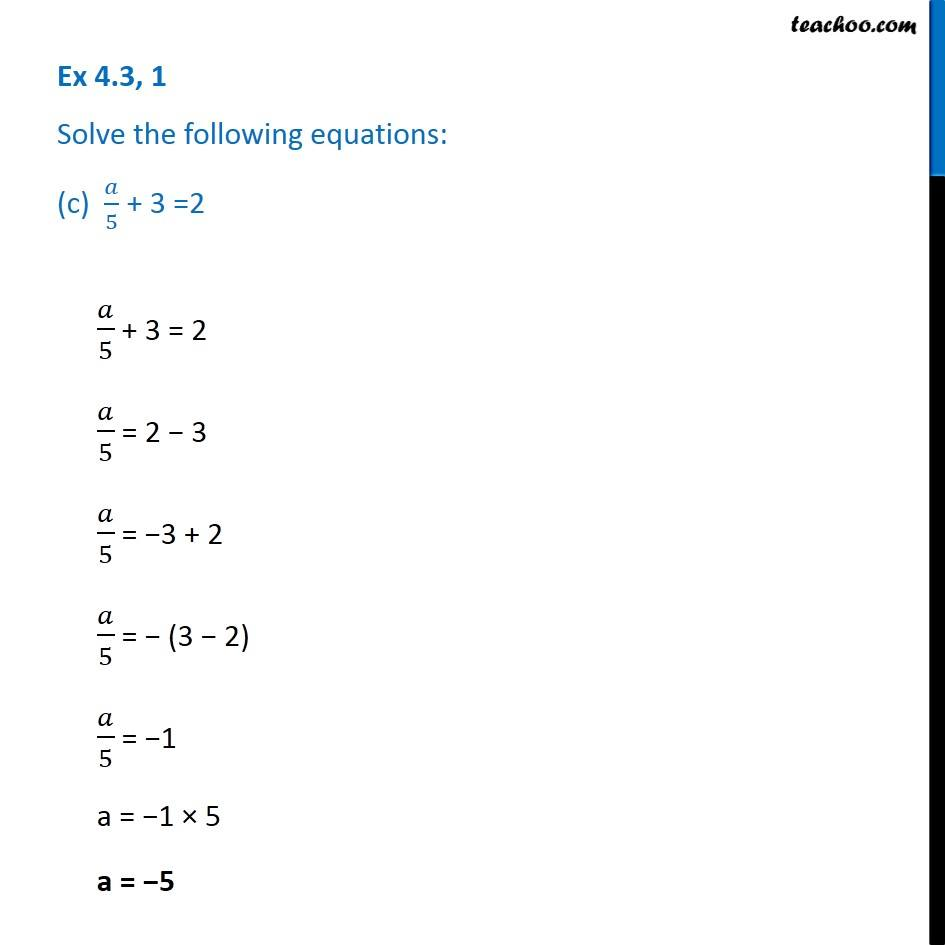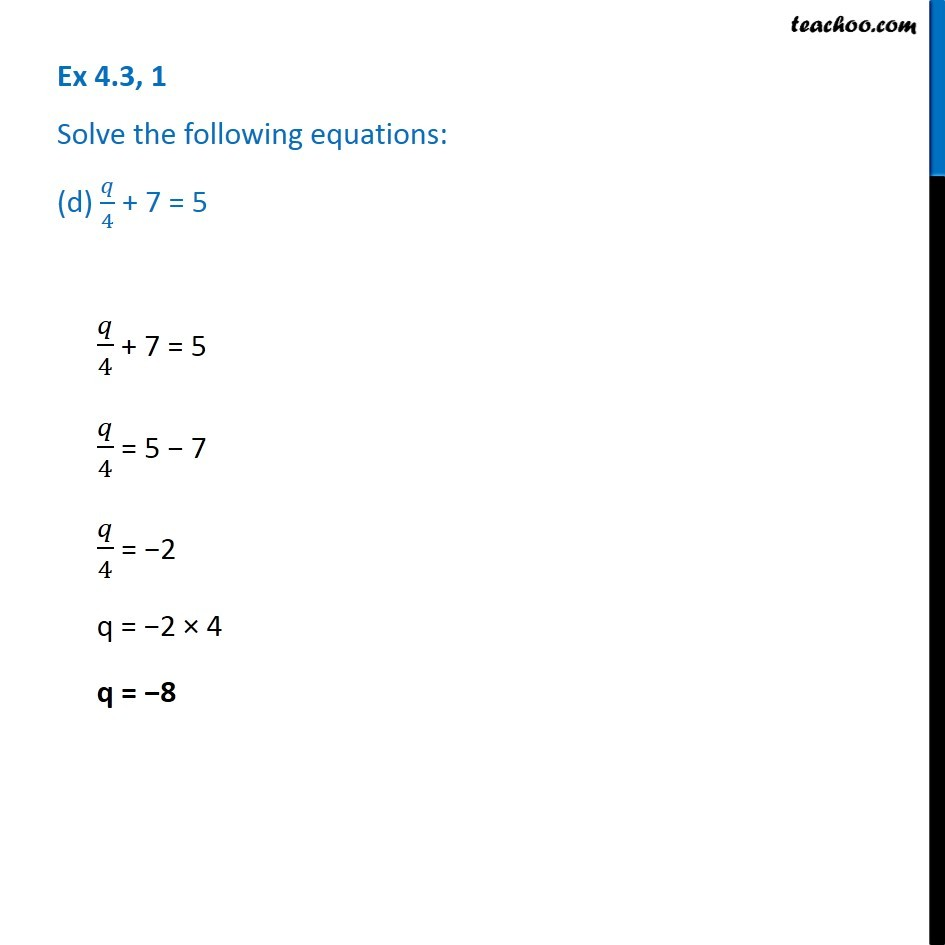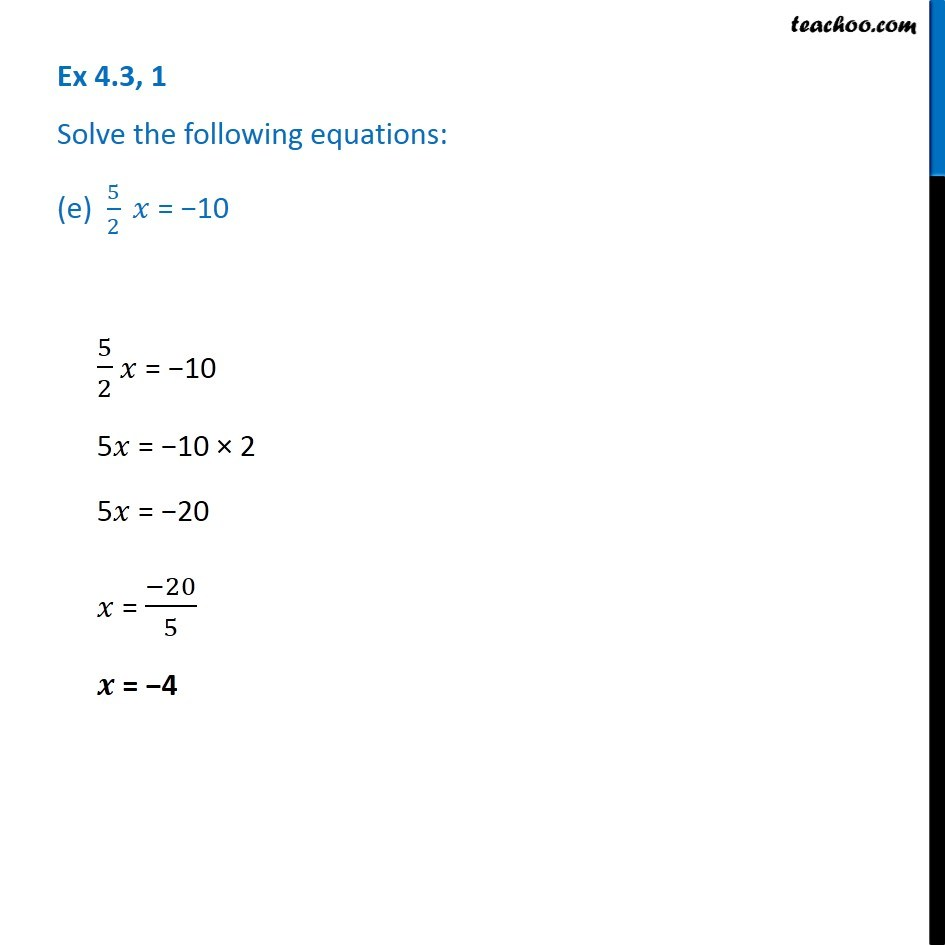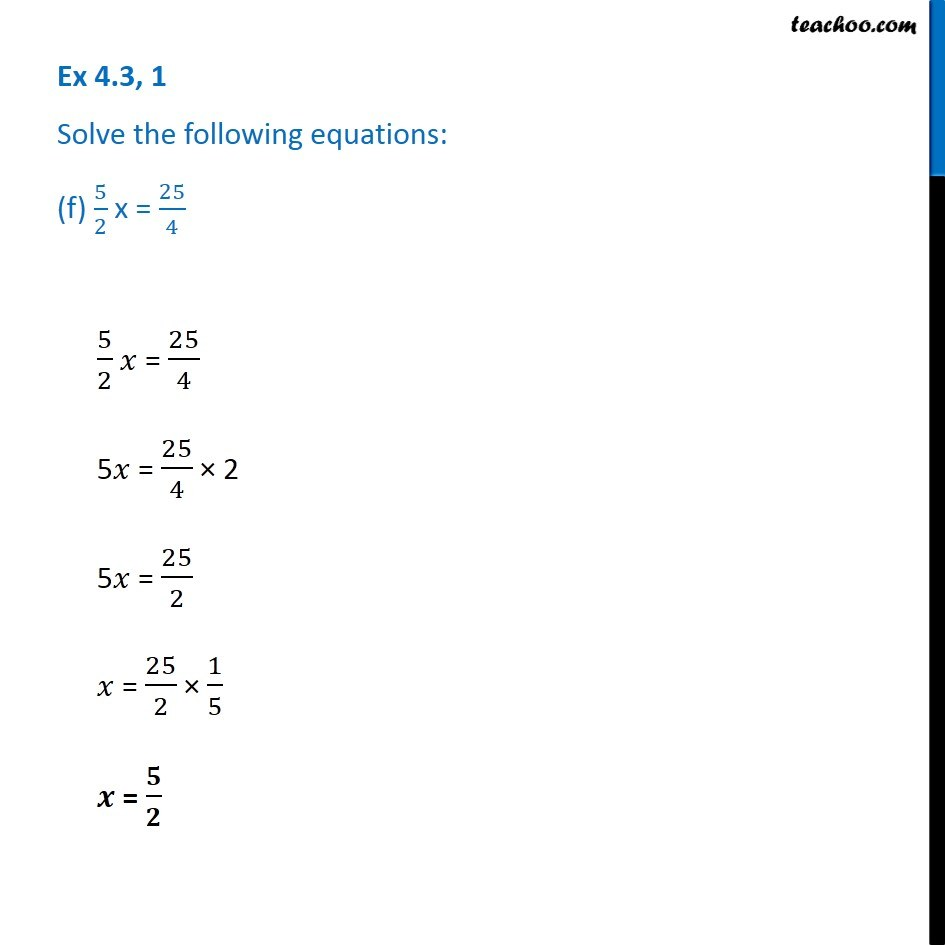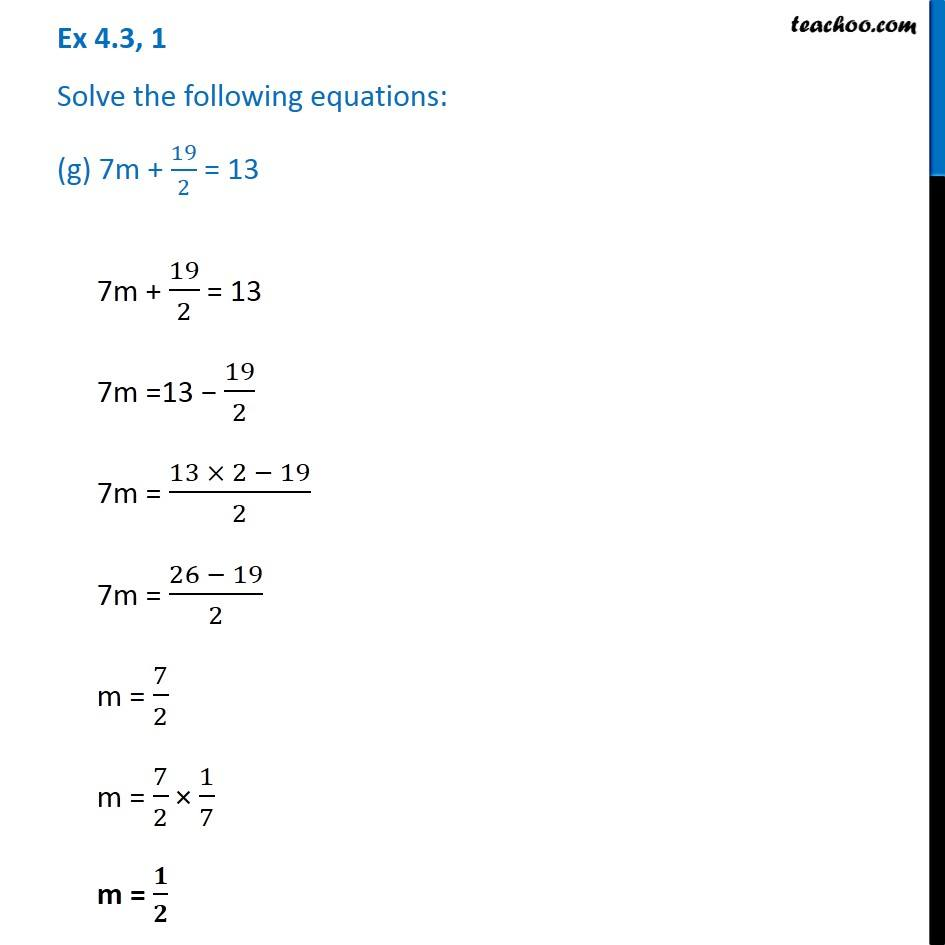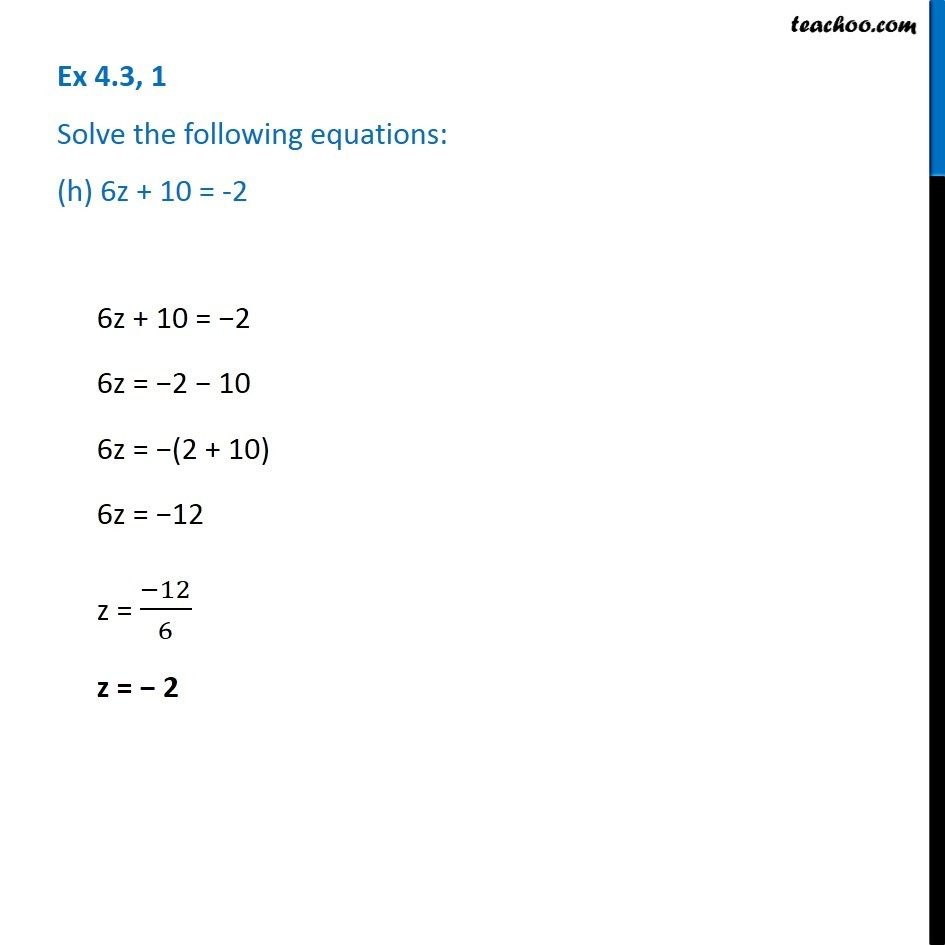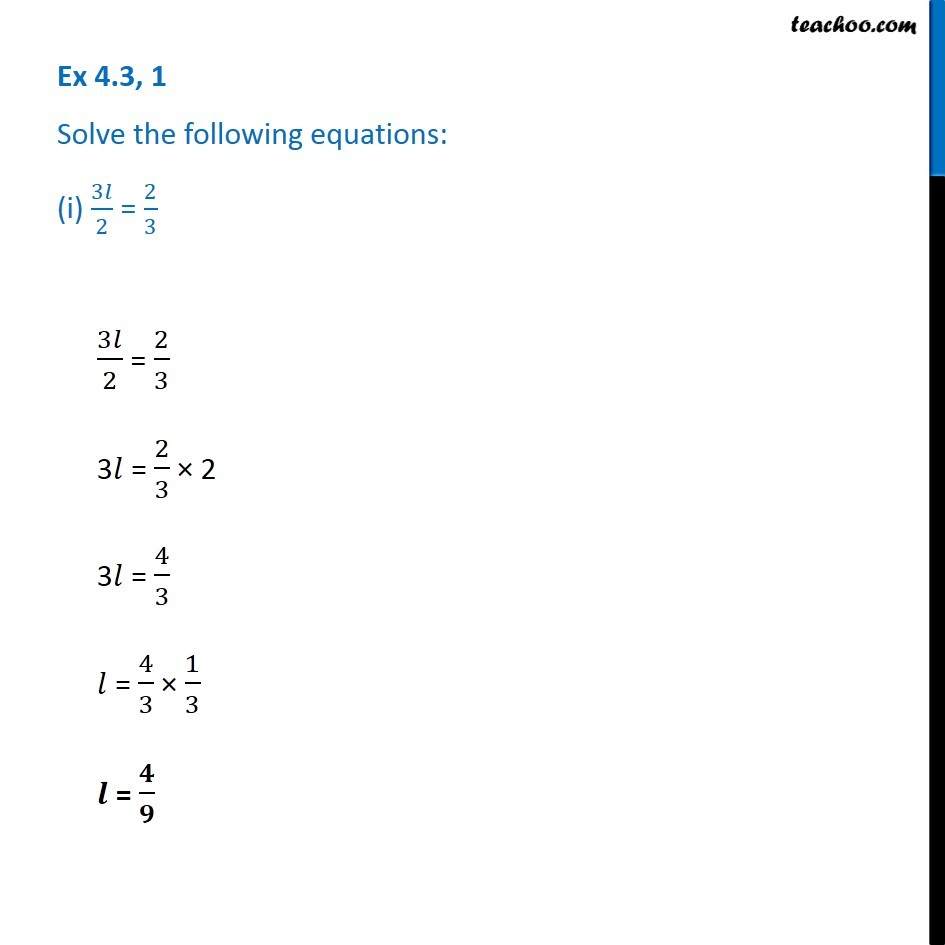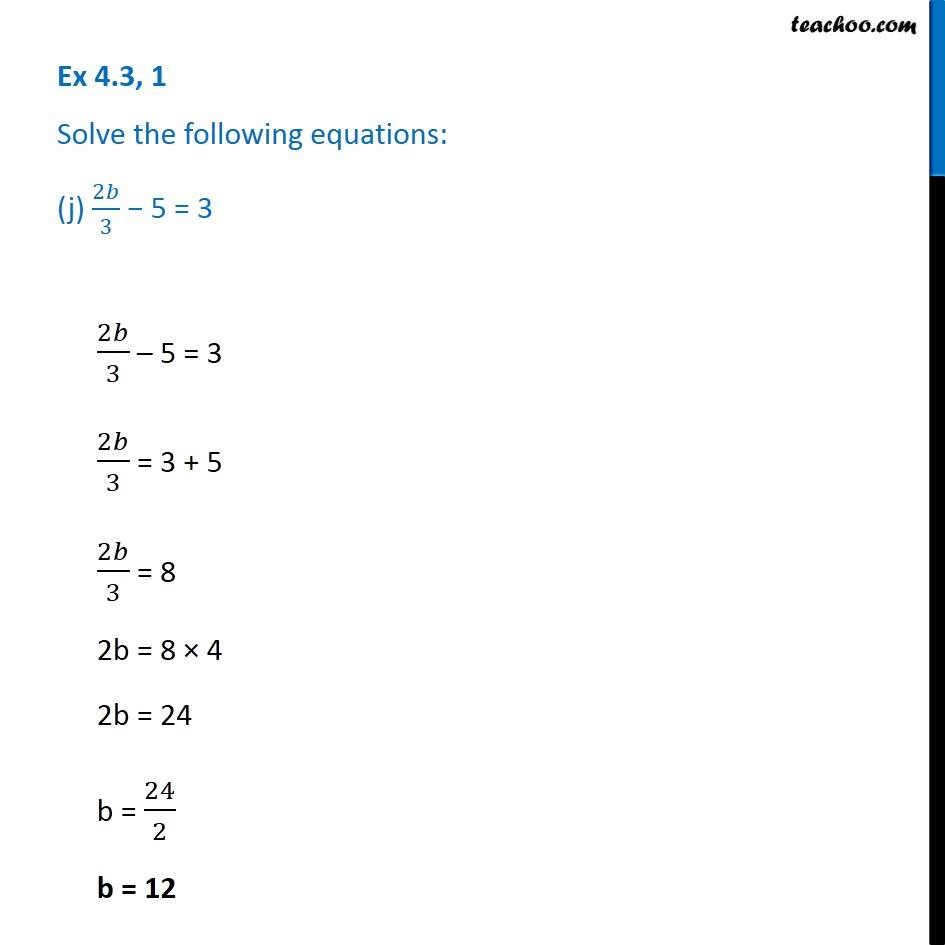Subscribe to our Youtube Channel - https://you.tube/teachoo

1. Chapter 4 Class 7 Simple Equations
2. Serial order wise
3. Ex 4.3

Transcript

Ex 4.3, 1 Solve the following equations: (a) 2y + 5/2 = 37/22y + 5/2 = 37/2 2y = 37/2 − 5/2 2y = (37 − 5)/2 2y = 32/2 2y = 16 y = 16/2 y = 8 Ex 4.3, 1 Solve the following equations: (b) 5t + 28 = 105t + 28 = 10 5t = 10 − 28 5t = −28 + 10 5t = − (28 − 10) 5t = −18 t = (−𝟏𝟖)/𝟓 Ex 4.3, 1 Solve the following equations: (c) 𝑎/5 + 3 =2𝑎/5 + 3 = 2 𝑎/5 = 2 − 3 𝑎/5 = −3 + 2 𝑎/5 = − (3 − 2) 𝑎/5 = −1 a = −1 × 5 a = −5 Ex 4.3, 1 Solve the following equations: (d) 𝑞/4 + 7 = 5𝑞/4 + 7 = 5 𝑞/4 = 5 − 7 𝑞/4 = −2 q = −2 × 4 q = −8 Ex 4.3, 1 Solve the following equations: (e) 5/2 𝑥 = −105/2 𝑥 = −10 5𝑥 = −10 × 2 5𝑥 = −20 𝑥 = (−20)/5 𝒙 = −4 Ex 4.3, 1 Solve the following equations: (f) 5/2 x = 25/45/2 𝑥 = 25/4 5𝑥 = 25/4 × 2 5𝑥 = 25/2 𝑥 = 25/2 × 1/5 𝒙 = 𝟓/𝟐 Ex 4.3, 1 Solve the following equations: (g) 7m + 19/2 = 137m + 19/2 = 13 7m =13 − 19/2 7m = (13 × 2 − 19)/2 7m = (26 − 19)/2 m = 7/2 m = 7/2 × 1/7 m = 𝟏/𝟐 Ex 4.3, 1 Solve the following equations: (h) 6z + 10 = -26z + 10 = −2 6z = −2 − 10 6z = −(2 + 10) 6z = −12 z = (−12)/6 z = − 2 Ex 4.3, 1 Solve the following equations: (i) 3𝑙/2 = 2/33𝑙/2 = 2/3 3𝑙 = 2/3 × 2 3𝑙 = 4/3 𝑙 = 4/3 × 1/3 𝒍 = 𝟒/𝟗 Ex 4.3, 1 Solve the following equations: (j) 2𝑏/3 − 5 = 32𝑏/3 – 5 = 3 2𝑏/3 = 3 + 5 2𝑏/3 = 8 2b = 8 × 4 2b = 24 b = 24/2 b = 12

Ex 4.3